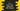# C++ and or && operator explanation with example## C++ ‘and’ or ’&&’ logical operator explanation with example:

and is a logical operator in C++. We can use only boolean values or expressions those returns boolean with logical operators. It operates on two operands.

This post will show you how and logical operator works in C++.

### Definition of and:

and logical operator is defined as:

``first_operand and second_operand``

we can also use && instead of and:

``first_operand && second_operand``

It evaluates to a boolean value. It will be True only if both of these operands are True. Else, it will be False.

It returns 1 for True and 0 for False.

### Example of &&:

Let me show you an example of &&:

``````#include <iostream>

using namespace std;

int main()
{
int i = 10;

cout << (i > 3 && i > 20) << endl;
cout << (i > 3 && i > 2) << endl;

return 0;
}``````

If you run this, it will print the below output:

``````0
1``````
• For the first one, it is printing 0 as this statement results false. i > 3 is true but i > 20 is false.
• For the second one, it prints 1 as it results true for both..

### Example with and

We can also replace && with and. It will print similar result.

``````#include <iostream>

using namespace std;

int main()
{
int i = 10;

cout << (i > 3 and i > 20) << endl;
cout << (i > 3 and i > 2) << endl;

return 0;
}``````

It will print:

``````0
1``````

### Example of and by taking user inputs:

Let’s write one program by taking user inputs. This program will ask the user to enter the age and it will print one message that the user is qualified if the age is greater than 18 and it is even.

``````#include <iostream>

using namespace std;

int main()
{
int age;

cout << "Enter your age: " << endl;
cin >> age;

if (age > 18 and age % 2 == 0)
{
cout << "You are qualified !!" << endl;
}
else
{
cout << "Please try again.." << endl;
}

return 0;
}``````

This program is reading the age value and put it in the variable age. By using age, it checks if it is greater than 18 and even or not. Based on that value, it prints a message to the user.

If you run this program, it will print output as like below:

``````Enter your age:
12